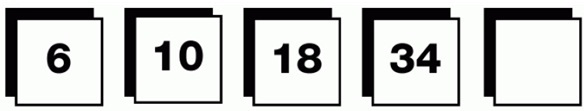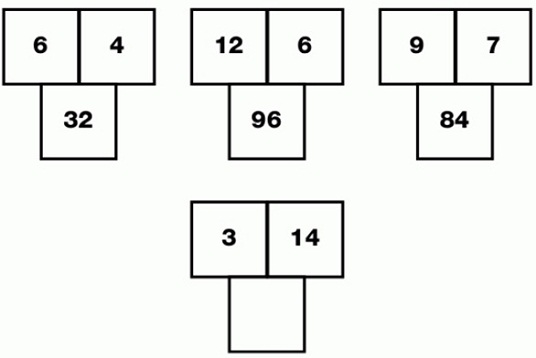##Numerical Puzzles Questions with Answers

1. Which number continues this sequence?Answer
 Ans = 66. Working from left to right, double the previous number and subtract 2.
2. Which number is missing from the bottom grid?Answer
 Ans = 56. In each diagram, divide the top left value by 3, multiply it by the top value, then multiply the answer by 4 to give the lower value.
12345

Page 1 of 5